### ISERROR Function Examples in Excel, VBA, & Google Sheets

This tutorial demonstrates how to use the Excel ISERROR Function in Excel to test if a cell results in an error.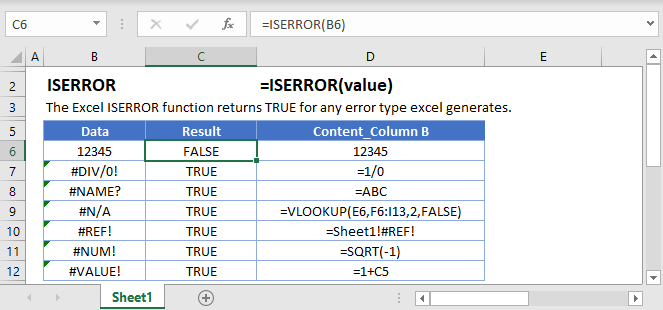## ISERROR Function Description:

The ISERROR Function Test if cell value is an error. Returns TRUE or FALSE.

To use the ISERROR Excel Worksheet Function, select a cell and type: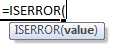(Notice how the formula inputs appear)

### ISERROR Function Syntax and Inputs:

value – The test value

AutoMacro - VBA Code Generator

## How to use the ISERROR Function

The ISERROR Function checks if a calculation results in an error.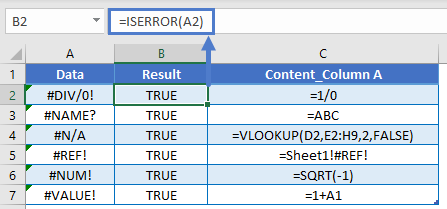### IF ISERROR Replaced by IFERROR

Prior to Excel 2007, the ISERROR Function was commonly used along with the IF Function to output a second calculation if the first calculation resulted in an error.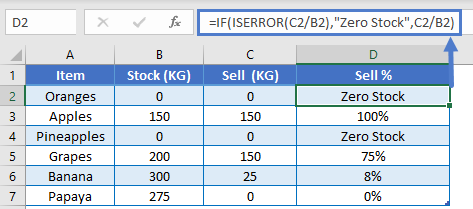However, in Excel 2007, the IFERROR Function was introduced, making the IF / ISERROR combo obsolete.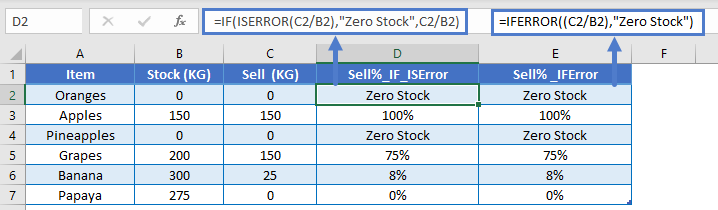### ISERR and ISNA

The ISERROR Function returns TRUE if any error occurs.

There are two other error checking “is” functions: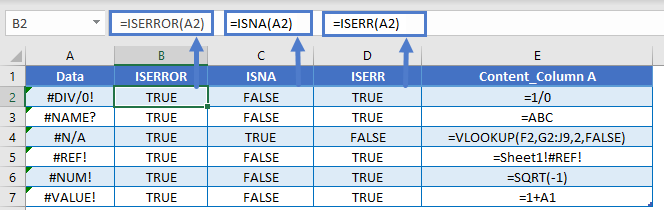Also, in addition to the IFERROR Function, there is the IFNA Function.

These functions are important because many formula errors are a result of bad formulas, but #N/A errors are often valid. For example, if you use the VLOOKUP Function and the lookup value is not found, the formula will return #N/A.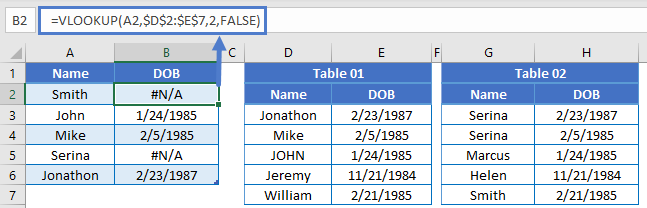By applying the IFNA Function to the VLOOKUP, you can handle valid #N/A errors, while not handling other formula errors (so you don’t overlook those other errors).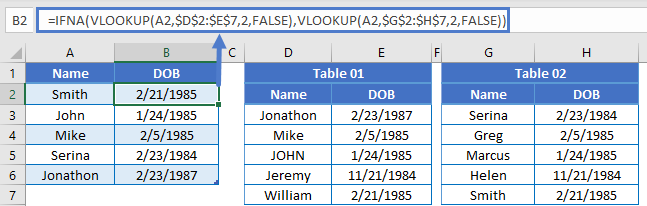### Other Logical Functions

Excel / Google Sheets contain many other logical functions to perform other logical tests. Here is a list:

IF / IS Functions
iferror
iserror
isna
iserr
isblank
isnumber
istext
isnontext
isformula
islogical
isref
iseven
isodd

## ISERROR in Google Sheets

The ISERROR Function works exactly the same in Google Sheets as in Excel: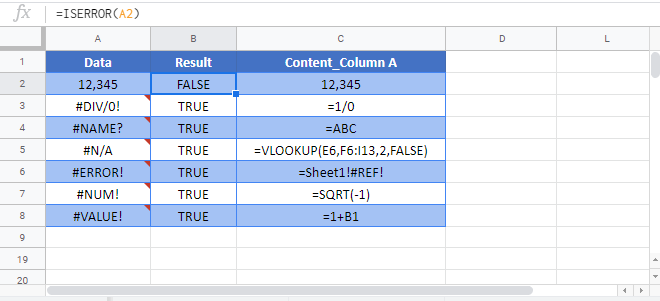## ISERROR Examples in VBA

You can also use the ISERROR function in VBA. Type:
`application.worksheetfunction.iserror(value)`
For the function arguments (value, etc.), you can either enter them directly into the function, or define variables to use instead.

Lets assume we have the following values in our worksheet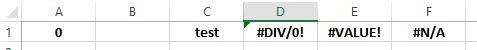We will issue the ISERROR function with VBA, for each of the above cells:

Return to the List of all Functions in Excel

## Excel Practice Worksheet

Practice Excel functions and formulas with our 100% free practice worksheets!

• Automatically Graded Exercises
• Learn Excel, Inside Excel!